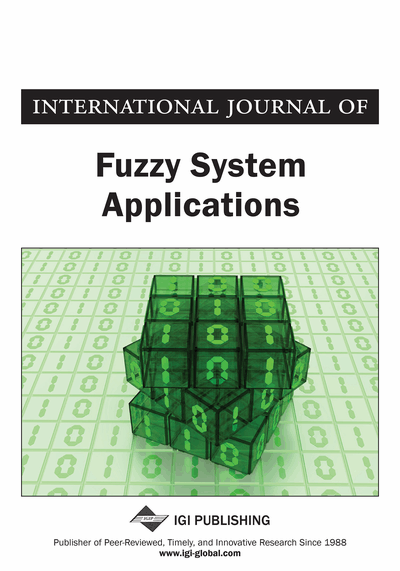# Hybrid Binomial Distribution

S. Sampath (University of Madras, Chennai, India)
DOI: 10.4018/ijfsa.2012100104
Available
\$29.50
No Current Special Offers

## Abstract

Buckley and Eslami (2003) introduced a discrete distribution, namely Fuzzy Binomial distribution, that is intended to suit situations wherein impreciseness and randomness coexist. Using the approach of Liu (2008), a hybrid (combination of imprecision and randomness) version of Binomial distribution is developed. With the help of genetic algorithms the process of computing the chance distributions, expectation and variance of the distribution that is developed in this paper are illustrated. Illustrative examples are given to justify the usefulness of the distribution.
Article Preview
Top

## Introduction

It is well known that probability distributions are models explaining the possible values of the underlying random variable along with the probabilities associated with them. For example, ifis a discrete random variable defined on an appropriate probability space taking values,, then the probability density function is shown in Figure 1.

whereanddefines probability distribution of. Examples of such distributions are Binomial, Poisson etc. It is pertinent to note that the probabilities’s will depend on certain parameters involved in the given probability distribution. For example, in the case of Binomial distribution whose probability distribution is explained by the model (1) the probabilities depend on the parameter. Similarly in the case of Poisson distribution whose probability distribution is given by (2) the probabilities depend on. Usually while evaluating such probabilities, values of the parametric values are expected to be known. Whenever values of such parametric values are not known, practitioners used to estimate them using some statistical estimation methods, like method of maximum likelihood, method of moments etc. For elementary ideas related to statistical estimation theory one can refer to Hogg et al. (2009). While this has been the practice of statisticians, the development of fuzzy theory due to Zadeh (1965, 1973) has led to alternative approaches. This theory of fuzzy sets can be used to effectively if appropriate membership functions for parameters involved in such distributions can be developed based on subject expertise. Membership functions of different types are available in the literature. Some examples are equipossible, triangular, trapezoidal and drum shaped membership functions. Buckley and Eslami (2003) considered Binomial Distribution where the parameteris treated as a fuzzy number. The process of evaluating probabilities for some specific choices along with the computational procedures related to the mean and variance of such a distribution are also discussed by them. In their entire discussion, interval valued quantities (based on-cuts) are obtained for probabilities, mean and variance of the distribution proposed by them. Practical situations where such an assumption is made have been considered by many including Jamkhaneh et al. (2008), and Jamkhaneh and Gildeh (2010). In this paper a hybrid version of Binomial distribution using a new theory that has been recently developed by Liu (2008), namely, chance theory is considered. The process of computing probabilities, mean and variance with the help of evolutionary algorithms is illustrated. A practical situation, which demands such a distribution in the Theory of Acceptance Sampling, is also considered. The fundamental difference between Buckley and Eslami (2003) and the present work is the values assumed by probabilities, mean and variance are fuzzy values in their approach whereas crisp values are obtained for such quantities in this work. The paper is organized as follows: The Uncertainty theory is briefly presented along with the definitions of mean and variance in the first section. The hybrid Binomial is discussed and computational aspects related to chance values, mean and variance are dealt with afterwards. Some illustrative examples are given in following that, in order to support the use of the chance distribution developed in this paper. The paper is concluded with some remarks.

## Complete Article List

Search this Journal:
Reset
Volume 12: 1 Issue (2023)
Volume 11: 4 Issues (2022)
Volume 10: 4 Issues (2021)
Volume 9: 4 Issues (2020)
Volume 8: 4 Issues (2019)
Volume 7: 4 Issues (2018)
Volume 6: 4 Issues (2017)
Volume 5: 4 Issues (2016)
Volume 4: 4 Issues (2015)
Volume 3: 4 Issues (2013)
Volume 2: 4 Issues (2012)
Volume 1: 4 Issues (2011)
View Complete Journal Contents Listing